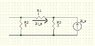# Node Voltage analysis

psycovic23
Find the equivalent resistance looking into terminals a-b for the network. Hint: first connect a 1A current source across terminals a and b. The solve the network by the node-voltage technique. The voltage across the current source is equal in value to the equivalent resistance.

So I've worked through the problem and figured out that the voltage at v1 (between 1 and 5 ohm resistor) is 5/3V and v2 (above 2 ohm resistor) is 1 V. I'm not entirely sure what the hint means so I don't know how to solve for the equivalent resistance. Any help would be appreciated. Thanks!

Note: I messed up in the picture. The current going through the resistor is only i_x, not 2i_x. The current source is (2 i_x).

#### Attachments

•circuit.jpg
8.4 KB · Views: 477